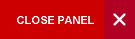## ECON3049: ECONOMETRICS

This is an introductory course, in the context of the simple two-variable model the classical linear regression model (CLRM) is thoroughly examined. Ordinary least squares estimators and the Gauss-Markov theorem in regression is presented. Tests of hypotheses, confidence intervals are covered. Problems that arise when the assumptions of the CLRM are relaxed are examined. The Basic Linear Regression Model Assumptions, Ordinary Least Squares, Properties of least Squares Estimators. Estimation of Economic Models, Testing of Economic Hypothesis Using Various Test Statistics; Multiple Co linearity, Seasonality, Dummy Variables, Auto Correlation and Specification Errors, Applicators of Econometric Methods to Caribbean examples.

Pre-requisites: (ECON2008 or ECON3031) or (MATH2404 & STAT2001) & (ECON2015 or MATH2410) (Anti-requisite: STAT3001)

Semester:

Lecturer: Dr. Andre Haughton

Position: Senior Lecturer

Office Hours: N/A

Office Location: Department Of Economics Main Block, Room 17